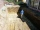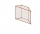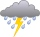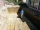# Volume of a prism problems

#### Number of problems found: 127

• The bodyThe body has dimensions of 2m 2dm and 10 cm. It weighs 28 kg. What is its density?
• Brick wallWhat is the weight of a solid brick wall that is 30 cm wide, 4 m long and 2 m high? The density of the brick is 1500 kg per cubic meter.
• The trenchCalculate how many cubic meters of soil needs to be removed from the excavation in the shape of an isosceles trapezoid, the top width is 3 meters, the lower width is 1.8 m, the depth of the excavation is 1 m, and the length is 20 m.The surface of the regular quadrilateral prism is 8800 cm2, the base edge is 20 cm long. Calculate the volume of the prism
• Trapezoidal prismCalculate the volume of a prism with a trapezoidal base with side a = 6dm, side c = 4 dm, height of the prism = 8dm. The height of the trapezoid is va = 3dm.
• Identical cubesFrom the smallest number of identical cubes whose edge length is expressed by a natural number, can we build a block with dimensions 12dm x 16dm x 20dm?
• Water tank300hl of water was filled into the tank 12 m long and 6 m wide. How high does it reach?
• RainGarden shape of a rectangle measuring 15 m and 20 m rained water up to 3 mm. How many liters of water rained on the garden?
• EmbankmentThe railway embankment 300 m long has a cross section of an isosceles trapezoid with bases of 14 m and 8 m. The trapezoidal arms are 5 m long. Calculate how much m3 of soil is in the embankment?
• Fire tank1428 hl of water is filled in a block-shaped fire tank with the edges of the base 12 m and 7 m. Calculate the content of water-wetted areas.
• The excavationThe excavation for the city sewer was 38 m long, 2.2 m wide, and 3 m deep. a) How many cubic meters of soil were dredged b) How many journeys had to be made by one car when removing the soil, if they loaded an average of 4.5 m of cubic soil on each journe
• Triangular prismThe base of the perpendicular triangular prism is a rectangular triangle with a hypotenuse of 10 cm and one leg of 8 cm. The prism height is 75% of the perimeter of the base. Calculate the volume and surface of the prism.Calculate the volume (V) and the surface (S) of a regular quadrilateral prism whose height is 28.6 cm and the deviation of the body diagonal from the base plane is 50°.
• Triangular prism,The regular triangular prism, whose edges are identical, has a surface of 2514 cm ^ 2 (square). Find the volume of this body in cm3 (l).
• AquariumTry to estimate the weight of the water in an aquarium 50cm long 30cm wide, when poured to a height of 25cm. Calculates the weight of the aquarium's water.
• PoolHow many hl of water is in a cuboid pool (a = 25m, b = 8m) if the area of the wetted walls is 279.2 m2?
• Uboid volumeCalculate the cuboid volume if the walls are 30cm², 35cm², 42cm²
• Swimming poolThe swimming pool has the shape of a block with dimensions of 70dm, 25m, 200cm. How many hl of water can fit into the pool?
• Body diagonalCalculate the volume of a cuboid whose body diagonal u is equal to 6.1 cm. Rectangular base has dimensions of 3.2 cm and 2.4 cm
• The cuboidThe cuboid is filled to the brim with water. The external dimensions are 95 cm, 120 cm, and 60 cm. The thickness of all walls and the bottom is 5 cm. How many liters of water fit into the cuboid?

Do you have an interesting mathematical word problem that you can't solve it? Submit a math problem, and we can try to solve it.

We will send a solution to your e-mail address. Solved examples are also published here. Please enter the e-mail correctly and check whether you don't have a full mailbox.

Please do not submit problems from current active competitions such as Mathematical Olympiad, correspondence seminars etc...

Tip: Our volume units converter will help you with the conversion of volume units.